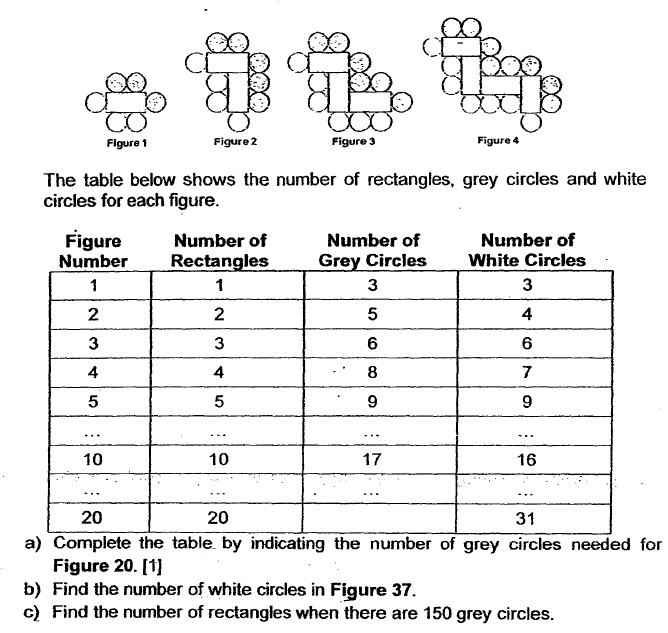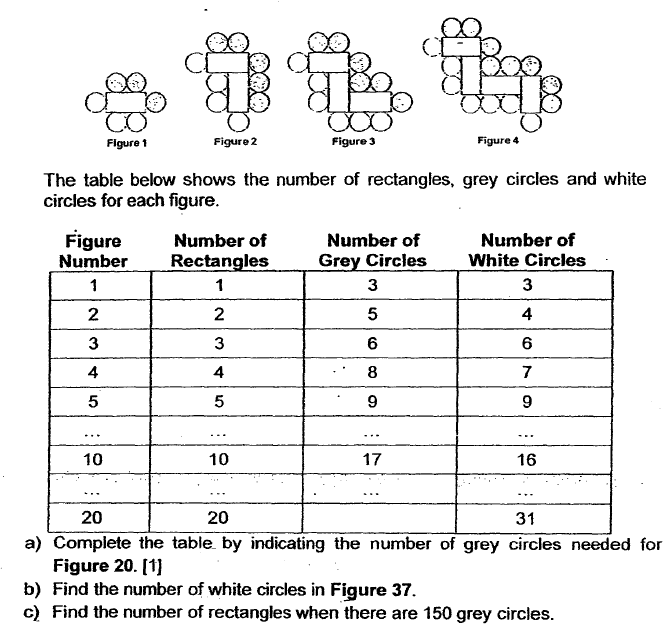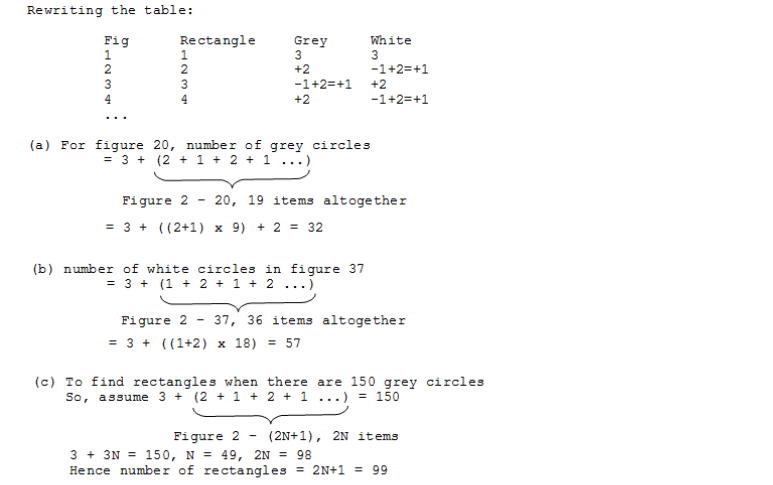# Questionplease kindly explain this kind of Qs . thank you in advance.

CS GO is one of the most popular multiplayer games at the moment.
csgo accounts

0 Replies 0 Likes

From observing:

1. No. of Grey Circles equalises with no. of White Circles when there is odd no. of rectangles.
2. Where there is even no. of rectangles, no. of Grey Circles is 1 more than White Circles
3. Add last column of total no. of circles, we see each additional rectangle creates 3 additional total circles
4. Let base no. of total circle be 3 circles.

1 rectangles –> 3+1 x 3 = 6

2 rectangles –> 3+2 x 3 = 9

3 rectangles –> 3+3 x 3 = 6

4 rectangles –> 3+4 x 3 = 15

Checking: 10 rectangles –> 3+10 x 3 = 33 so 17 grey and 16 white circles

Solving:

20 rectangles –> 3+20 x 3 = 63, so 32 grey and 31 white circles (ans. 32 grey- can also deduce based on observation #2 above)

37 rectangles –> 3+37 x 3 = 114, so 57 grey and 57 white circles (ans. 57 white)

working backwards: 150 grey and 150 white circles –> 300 = 3+ 99 x (ans. 99 rectangles)

Checking: 150 grey and 149 white circles –> 299 = 3+ 98.6 x (cannot solve for rectangles)

HI, SAHMOM, thank you so much for your detail explanation. appreciate .

My working for part c,

no. of greys = 150

No.of white = 150                   total circles = 300

(No. of rec + 1) * 3 = 300
3 No.of rec + 3 = 300
No of rec = 99

a)

fig : number of grey circles

2 : 5

4 : 8

6 : 11

20 : 5 + [(20 – 2)/2] x 3  = 32

b)

fig : number of white circles

1 : 3

3 : 6

5 : 9

37 : 3 + [(37 – 1)/2] x 3  = 57

c)

150 – 3 = 147

2 + 1 = 3

(147/3) x 2 + 1 = 99

Ans : a) 32 grey circles; b) 57 white circles; c) 99 rectangles.

0 Replies 0 LikesObservations:

a.  Number of rectangles = Figure number

b.  Odd Figure number and grey circle/white circles:

Grey/White circles  = (nth odd number) x 3.

So, the number of grey/white circles are multiples of 3 for Odd Figure number.

c.  Even figure number and grey /white circles:

number of white circle = (number of white circles in preceding Odd figure number) + 1,

number of grey circles = (number of grey circles in preceding Odd figure number + 2)

= (number of white circles in SAME figure number +1)

a) Number of grey circles in Figure 20= 31 + 1 = 32

b) 37 = (37+1)/2 th odd number = 19th odd number

Number of white/grey circles = 19 x 3 = 57

c) 150 is a multiple of 3,  so the Figure number is ODD.

150 / 3 = 50

50th odd number = (50×2)-1 = 99

Figure number / number of rectangles = 99

0 Replies 0 Likes0 Replies 0 Likes